Courses

# Principal Stress And Strain - MCQ Test 2

## 30 Questions MCQ Test RRB JE for Mechanical Engineering | Principal Stress And Strain - MCQ Test 2

Description
This mock test of Principal Stress And Strain - MCQ Test 2 for Mechanical Engineering helps you for every Mechanical Engineering entrance exam. This contains 30 Multiple Choice Questions for Mechanical Engineering Principal Stress And Strain - MCQ Test 2 (mcq) to study with solutions a complete question bank. The solved questions answers in this Principal Stress And Strain - MCQ Test 2 quiz give you a good mix of easy questions and tough questions. Mechanical Engineering students definitely take this Principal Stress And Strain - MCQ Test 2 exercise for a better result in the exam. You can find other Principal Stress And Strain - MCQ Test 2 extra questions, long questions & short questions for Mechanical Engineering on EduRev as well by searching above.
QUESTION: 1

Solution:
QUESTION: 2

Solution:
QUESTION: 3

### Principal strains at a point are 100 x 10-6 and -200 x 10-6 . What is the maximum shear strain at the point?

Solution: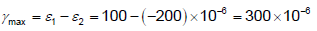don' t confusewithMaximumShear stress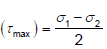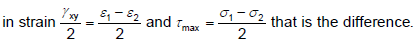QUESTION: 4

The number of strain readings (using strain gauges) needed on a plane surface to determine the principal strains and their directions is:

Solution:

Three strain gauges are needed on a plane surface to determine the principal strains and their directions.

QUESTION: 5

Assertion (A): Mohr's construction is possible for stresses, strains and area moment of inertia.

Reason (R): Mohr's circle represents the transformation of second-order tensor.

Solution:
QUESTION: 6

On a plane, resultant stress is inclined at an angle of 45o to the plane. If the normal stress is 100 N /mm2, the shear stress on the plane is:

Solution: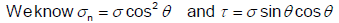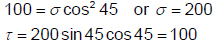QUESTION: 7

The complementary shear stresses of intensity τ are induced at a point in the material, as shown in the figure. Which one of the following is the correct set of orientations of principal planes with respect to AB?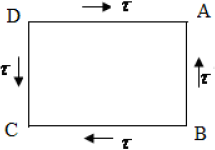Solution:

It is a case of pure shear so principal planes will be along the diagonal.

QUESTION: 8

A body is subjected to a pure tensile stress of 100 units. What is the maximum shear produced in the body at some oblique plane due to the above?

Solution: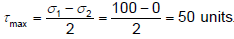QUESTION: 9

A material element subjected to a plane state of stress such that the maximum shear stress is equal to the maximum tensile stress, would correspond to

Solution: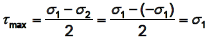QUESTION: 10

A solid circular shaft of diameter 100 mm is subjected to an axial stress of 50 MPa. It is further subjected to a torque of 10 kNm. The maximum principal stress experienced on the shaft is closest to

Solution:

Shear Stress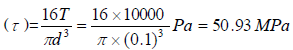Maximum principal Stress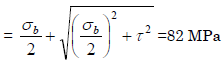QUESTION: 11

The maximum principle stress for the stress state shown in the figure is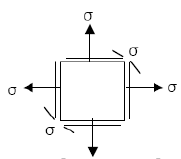Solution: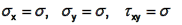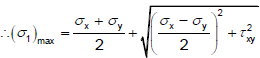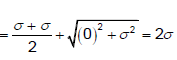QUESTION: 12

In a Mohr's circle, the radius of the circle is taken as:

Where, σx and σy are normal stresses along x and y directions respectively and τxy is the shear stress.

Solution: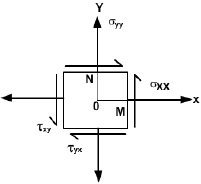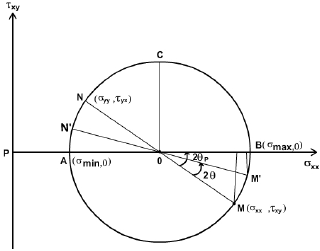QUESTION: 13

The Mohr's circle of plane stress for a point in a body is shown. The design is to be done on the basis of the maximum shear stress theory for yielding. Then, yielding will just begin if the designer chooses a ductile material whose yield strength is: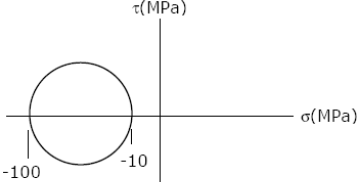Solution: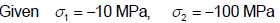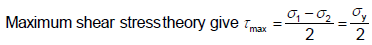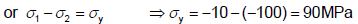QUESTION: 14

Determine the directions of maximum and minimum principal stresses at the point “P” from the Mohr's circle

Solution:

From the Mohr‟s circle it will give all directions

QUESTION: 15

Which one of the following Mohr‟s circles represents the state of pure shear?

Solution:
QUESTION: 16

Assertion (A): If the state at a point is pure shear, then the principal planes through that point making an angle of 45° with plane of shearing stress carries principal stresses whose magnitude is equal to that of shearing stress.

Reason (R): Complementary shear stresses are equal in magnitude, but opposite in direction.

Solution:
QUESTION: 17

When a component is subjected to axial stress the normal stress σn is maximum, if cos θ is _______ . (σnxCos2θ)

1. maximum
2. minimum
3. always one
4. always zero

Solution:
QUESTION: 18

An elastic material of Young‟s modulus E and Poisson‟s ratio ν is subjected to a compressive stress of σ1 in the longitudinal direction. Suitable lateral compressive stress σ 2 are also applied along the other two each of the lateral directions to half of the magnitude that would be under σ1 acting alone. The magnitude of σ2 is

Solution:
QUESTION: 19

If the principal stresses and maximum shearing stresses are of equal numerical value at a point in a stressed body, the state of stress can be termed as

Solution:
QUESTION: 20

A piece of material is subjected, to two perpendicular tensile stresses of 70 MPa and 10 MPa. The magnitude of the resultant stress on a plane in which the maximum shear stress occurs is

Solution:
QUESTION: 21

Principal stresses at a point in plane stressed element are σx = σ= 500 kg/cm2 . Normal stress on the plane inclined at 45o to x-axis will be:

Solution:

When stresses are alike, then normal stress σn on plane inclined at angle 45° is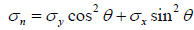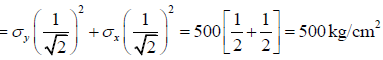QUESTION: 22

At a point in two-dimensional stress system σx = 100 N/mm2, σy = τxy = 40 N/mm2. What is the radius of the Mohr circle for stress drawn with a scale of: 1 cm = 10 N/mm2?

Solution: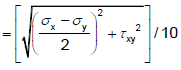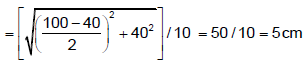QUESTION: 23

Assertion (A): Mohr's circle of stress can be related to Mohr's circle of strain by some constant of proportionality

Reason (R): The relationship is a function of yield stress of the material.

Solution:
QUESTION: 24

The principal strains at a point in a body, under biaxial state of stress, are 1000 ×10–6 and –600 × 10–6. What is the maximum shear strain at that point?

Solution: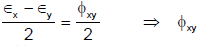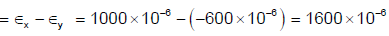QUESTION: 25

Which of the following stresses can be determined using Mohr's circle method?

Solution:
QUESTION: 26

Normal stresses of equal magnitude p, but of opposite signs, act at a point of a strained material in perpendicular direction. What is the magnitude of the resultant normal stress on a plane inclined at 45° to the applied stresses?

Solution: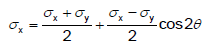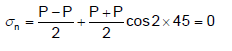QUESTION: 27

A point in two-dimensional stress state, is subjected to biaxial stress as shown in the above figure. The shear stress acting on the plane AB  is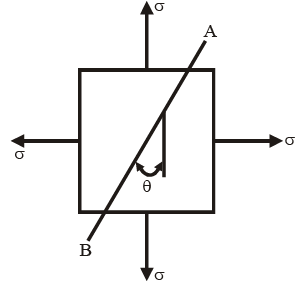Solution: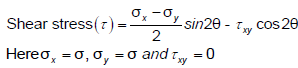QUESTION: 28

What are the normal and shear stresses on the 45o planes shown?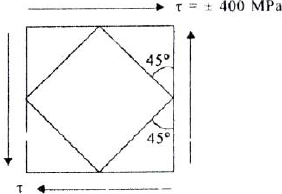Solution:
QUESTION: 29

Consider the Mohr's circle shown above: What is the state of stress represented by this circle?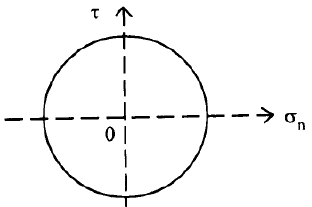Solution:

It is a case of pure shear. 1 2 Just put σ1 = - σ2

QUESTION: 30

State of stress in a plane element is shown in figure I. Which one of the following figures -II is the correct sketch of Mohr's circle of the state of stress?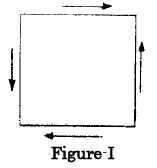Solution: